In this tutorial, we will learn about the N-bit Parallel Adders (4-bit Binary adders and Subtractors) in Digital Electronics. By Saurabh Gupta Last updated : May 11, 2023

Two n+1-bit binary number A and B of the form,

```A:  An An-1 An-2 ... A3 A2 A1 A0 (Augend)
B:  Bn Bn-1 Bn-2 ... B3 B2 B1 B0 (Addend)
```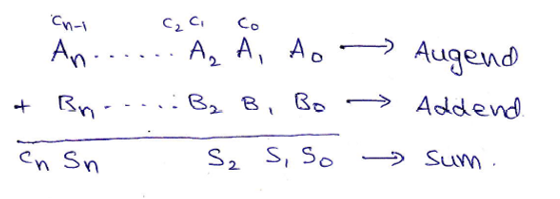Block diagram and Logic circuit diagram of a Parallel Binary adder can be given as,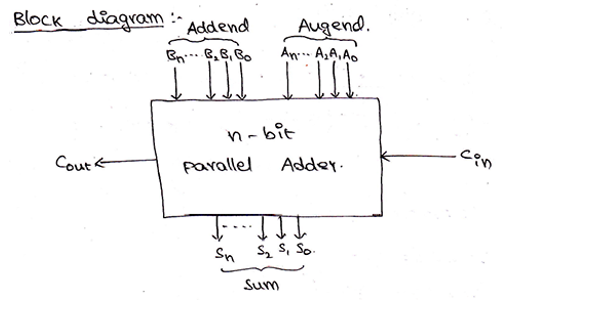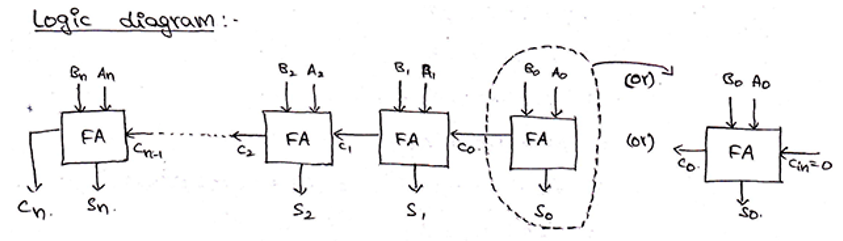From the above-provided logic, we need 4 full adders connected together to add 4-bit binary numbers. For 4-bit binary numbers A and B of the form,

A: A3 A2 A1 A0 and B: B3 B2 B1 B0, its sum can be obtained as,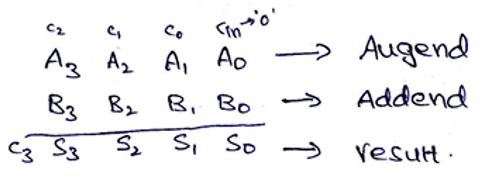Block diagram and Logic circuit diagram of a 4-bit Binary adder can be given as,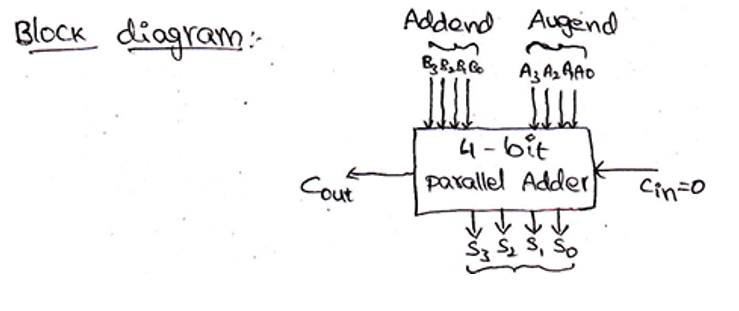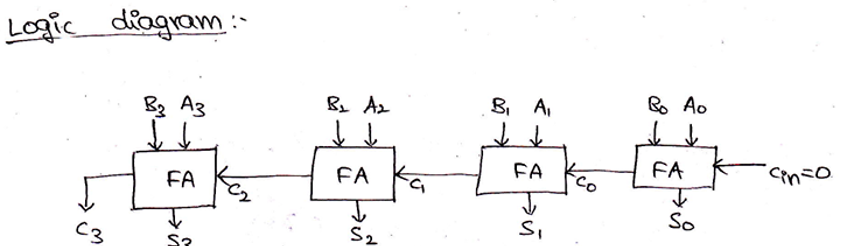## 4-Bit Binary Subtractor

We already know that two numbers A (Minuend) and B (Subtrahend) can be subtracted using 2s complement method, where,

```A – B   = A + 2s complement of B
= A + 1s complement of B + 1
```

Thus, 4-bit binary numbers A and B can subtract as,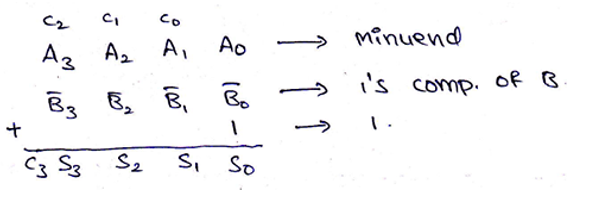And the logic circuit for the same can be drawn as,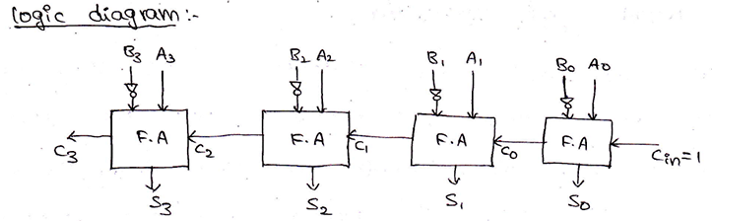Parallel Adder/Subtractor using a single circuit can be also designed using a Mod bit (M), where mod bit M decides whether the circuit will act as an adder or a subtractor. When M=0, then the circuit acts as an adder and when M=1, then the circuit acts as a subtractor. The circuit for the same can be drawn as,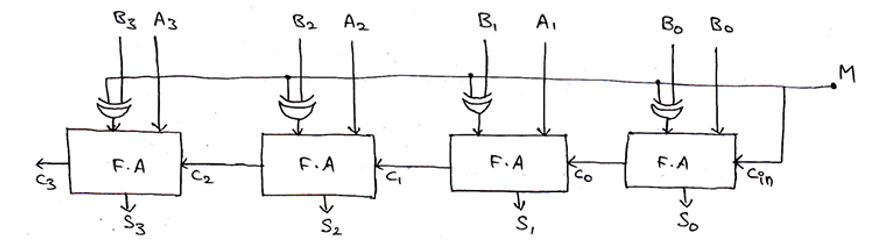```When M=0,
output  = A3 A2 A1 A0 + B3 B2 B1 B0 + Cin          [Since, A⊕0 = A]
= A3 A2 A1 A0 + B3 B2 B1 B0 + 0
= A + B
```

Thus, circuit acts as a 4-bit binary adder.

```When M=1,
output  = A3 A2 A1 A0 + B3 B2 B1 B0 + Cin
= A3 A2 A1 A0 + B3 B2 B1 B0 + 1
= A + B + 1
= A – B
```

Thus, circuit acts as a 4-bit binary subtractor